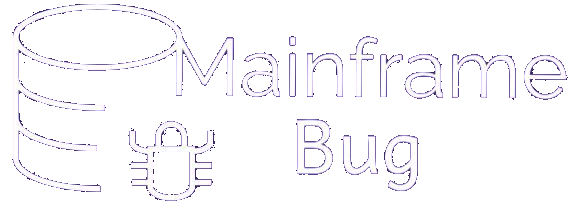## COBOL Quiz

### COBOL Quiz 1-10

• Q1 - The maximum number of digits in a numeric item is
• A - 16
• B - 17
• C - 18
• D - 20
• Answer : C - 18
• Q2 - What is 88 level used for
• A - For RENAMES clause.
• B - For condition names
• C - For REDEFINES clause.
• D - None of above
• Answer : B - For condition names
• Q3 - Which of the following is not a VALID COBOL figurative constant?
• A - ZERO
• B - ZEROS
• C - ONES
• D - QUOTES
• Answer : C - ONES
• Q4 - What is 77 level used for ?
• A - For RENAMES clause.
• B - For REDEFINES clause.
• C - For Elementary level item
• D - None of above
• Answer : C - For Elementary level item
• Q5 - Which one of the following is NOT correct?
• A - ADD A, B GIVING C, D
• B - ADD CORRESPONDING GROUP-VAR1 TO GROUP-VAR2.
• C - ADD A TO B GIVING C.
• D - ADD A TO B, C TO D.
• Answer : D - ADD A TO B, C TO D.
• Q6 - Can the OCCURS clause be at the 01 level?
• A - Yes
• B - No
• Answer : B - No
• Q7 - How many bytes S9(09) COMP will occupy ?
• A - 10 bytes
• B - 9 bytes
• C - 4 bytes
• D - 3 bytes
• Answer : C - 4 bytes
• Q8 - An index can be manipulated by a MOVE statement
• A - True
• B - False
• Answer : B - False
• Q9 - In an EVALUATE statement, can I give a complex condition on a when clause
• A - Yes
• B - No
• Answer : A - Yes
• Q10 - What is the maximum value that can be stored in S9(8) COMP?
• A - 9999999999
• B - 99999999
• C - 9999
• D - 999999999
• Answer : B - 99999999